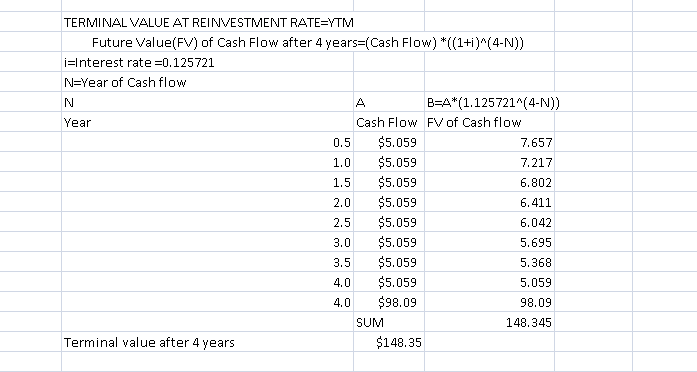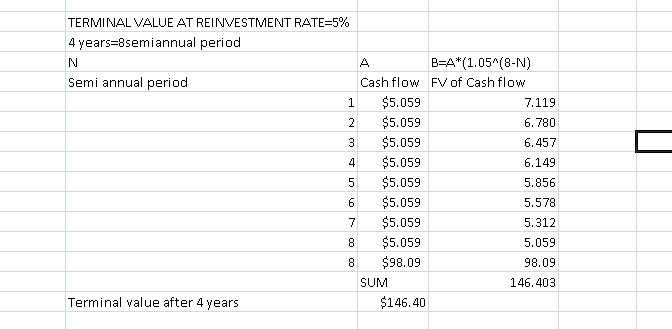# Consider a bond with market value \$92.37 (face value = \$100) and a coupon of \$5.059 dollars paid ...

Consider a bond with market value \$92.37 (face value = \$100) and a coupon of \$5.059 dollars paid every six months (Semi-annually - December and June). The time to maturity is 10 semiannual periods. Assume today is January 1st, 2015. Therefore, the face value of the bond will be repaid on December 2019.

Upload your spreadsheet on the course website (you might want to use the spreadsheet “11 Bond Yield and Duration Example” that we analyzed in class as a starting point for your calculations) and provide the answers to the following questions on paper.

1. Compute the annual YTM of the bond, using Excel Solver (note: the question asks for the annual YTM, not the semiannual. If you compute the semiannual YTM, you must compound it using the effective annual rate formula). [1.25 points]
2. Compute the duration of the bond. Please say clearly what unit you are using, e.g. duration is N years or duration is N semiannual periods. [1 point]
3. Four years from now, if the YTM remains constant, what will be the resale price of the bond? (HINT: since time to maturity is now 10 semiannual periods, the bond will have a time to maturity of two semiannual periods, four years from now; the cash-flow that you must consider to compute the price are two coupon payments, and the face value repaid at maturity). [1.25 points]
4. If the reinvestment rate is equal to the YTM, what is the terminal value of the portfolio after 4 years? (HINT: the terminal value of the portfolio is the sum of the coupons reinvest at the reinvestment rate, plus the revenues from selling the bond 4 years from now) [1.25 points]
5. If the reinvestment rate is now 5% (semiannual), what is the terminal value of the portfolio after 4 years? Why is it almost the same as your previous answer? Why isn’t it exactly the same as your previous answer? (HINT: the change in the reinvestment rate affects both the value of the reinvest coupons, and the resale price of the bond). [1.25 points]

 CALCULATION OF YTM Pv Current Market Value \$92.37 Nper Number of semi annual period to maturity 10 Pmt Semi annual coupon payment \$5.059 Fv Redemption payment at end of 10 periods \$100 RATE Semi annual Yield to maturity 6.10% (Using RATE function of excel with Nper=10,Pmt=5.059,Pv=-92.37,Fv=100) Annual YTM =((1+0.0610)^2)-1 0.125721 Annual YTM in percentage 12.5721% DURATION OF BOND N A B=A/(1.125721^N) C=A*N D=C/(1.125721^N) Annual Period Cash Flow Present Value Cash flow* PV of (cash (PV)of Cash flow Period flow*Period) 0.5 \$5.059 4.768 \$2.530 2.384 1.0 \$5.059 4.494 \$5.059 4.494 1.5 \$5.059 4.236 \$7.589 6.353 2.0 \$5.059 3.992 \$10.118 7.984 2.5 \$5.059 3.763 \$12.648 9.406 3.0 \$5.059 3.546 \$15.177 10.639 3.5 \$5.059 3.342 \$17.707 11.698 4.0 \$5.059 3.150 \$20.236 12.601 4.5 \$5.059 2.969 \$22.766 13.361 Cashflow= (100+5.059) 5.0 \$105.059 58.114 \$525.295 290.569 SUM 92.37 369.49 l Bond Duration in years 3.999926 (369.49/92.37) RESALE PRICE AFTER 4 YEARS CF PV=CF/(1.125721^N) Annual Period after 4 years Cash flow PV of cash Flow 0.5 \$5.059 4.768 Cashflow= (100+5.059) 1 \$105.059 93.326 SUM 98.09 Resale Price After 4 years \$98.09##### Add Answer of: Consider a bond with market value \$92.37 (face value = \$100) and a coupon of \$5.059 dollars paid ...
More Homework Help Questions Additional questions in this topic.